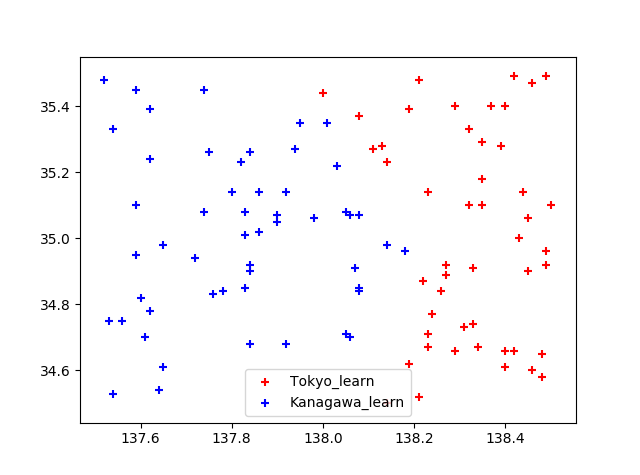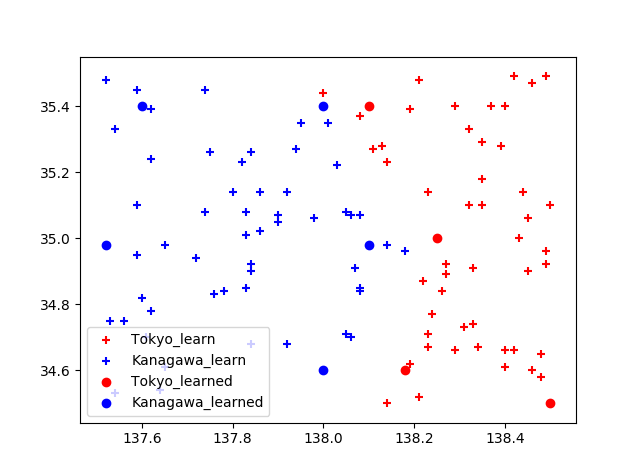# Introduction

① https://qiita.com/yohiro/items/04984927d0b455700cd1 ② https://qiita.com/yohiro/items/5aab5d28aef57ccbb19c ③ https://qiita.com/yohiro/items/cc9bc2631c0306f813b5 Continued

--Reference materials: Udemy Everyone's AI course Artificial intelligence and machine learning learned from scratch with Python --Issue setting: Given latitude and longitude, create a program to determine whether it belongs to Tokyo or Kanagawa. --Judgment method: Judgment is made by a neural network with 2 input layers, 2 intermediate layers, and 1 output layer.

# Machine learning with backpropagation

The weight of neurons in the output layer is adjusted based on the error between the output and the correct answer. Moreover, the weight of the neuron in the intermediate layer is adjusted based on the weight of the neuron in the output layer.

# Intermediate layer-Adjusting the weight of the output layer

Intermediate layer-The weight adjustment of the output layer can be obtained by the following formula.

\delta_{mo} = (Output value-Correct answer value) \derivatives of the times output\\
Correction amount= \delta_{mo} \times middle layer value\times learning coefficient


## Differentiation of sigmoid function

Regarding the "derivative of output" in the above equation, the derivative of the sigmoid function $f (x) = \ frac {1} {1 + e ^ x}$ used in the activation function of the neural network this time is as follows. can get.

f(x)' = f(x)\cdot(1-f(x))


Reference: https://qiita.com/yosshi4486/items/d111272edeba0984cef2

## Source code

class NeuralNetwork:
#Input weight
w_im = [[1.0, 1.0], [1.0, 1.0], [1.0, 1.0]]  # [[i1-m1, i1-m2], [i2-m1, i2-m2], [bias1-m1, bias1-m2]]
w_mo = [1.0, 1.0, 1.0]  # [m1-o, m2-o, bias2-0]
#Declaration of each layer
input_layer = [0.0, 0.0, 1.0] # i1, i2, bias1
middle_layer = [Neuron(), Neuron(), 1.0] # m1, m2, bias2
ouput_layer = Neuron() # o

def learn(self, input_data):

#Output value
output_data = self.commit([input_data, input_data])
correct_value = input_data
#Learning coefficient
k = 0.3

#Output layer → Intermediate layer
delta_w_mo = (correct_value - output_data) * output_data * (1.0 - output_data)
old_w_mo = list(self.w_mo)
self.w_mo += self.middle_layer.output * delta_w_mo * k
self.w_mo += self.middle_layer.output * delta_w_mo * k
self.w_mo += self.middle_layer * delta_w_mo * k


# Input layer-Adjusting intermediate layer weights

The weight adjustment of the input layer-intermediate layer can be obtained by the following equation. By adjusting the weight of the front neuron based on the adjustment result of the rear neuron, it is possible to adjust any number of layers.

\delta_{im} = \delta_{mo} \times Intermediate output weight\times Differentiation of intermediate layer output\\
Correction amount= \delta_{im} \times Input layer value\times learning coefficient


## Source code

class NeuralNetwork:
...
#Middle layer → Input layer
delta_w_im = [
delta_w_mo * old_w_mo * self.middle_layer.output * (1.0 - self.middle_layer.output),
delta_w_mo * old_w_mo * self.middle_layer.output * (1.0 - self.middle_layer.output)
]
self.w_im += self.input_layer * delta_w_im * k
self.w_im += self.input_layer * delta_w_im * k
self.w_im += self.input_layer * delta_w_im * k
self.w_im += self.input_layer * delta_w_im * k
self.w_im += self.input_layer * delta_w_im * k
self.w_im += self.input_layer * delta_w_im * k


# Learning results

Prepare the following as training dataIf you throw the following data into the neural network trained above ...

data_to_commit = [[34.6, 138.0], [34.6, 138.18], [35.4, 138.0], [34.98, 138.1], [35.0, 138.25], [35.4, 137.6], [34.98, 137.52], [34.5, 138.5], [35.4, 138.1]]


You can see that it is possible to determine whether it is classified as Tokyo or Kanagawa.